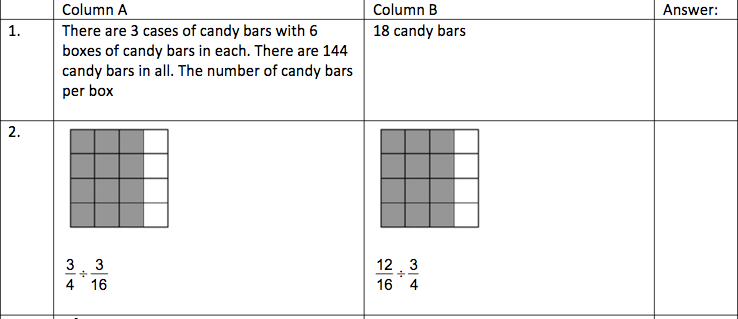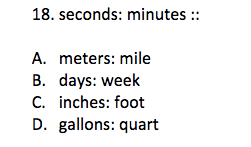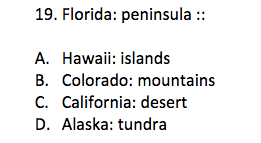Start Now with 100 Free Questions

# SCAT 4th – 5th Grade Practice Test

## Test Structure

The SCAT is divided into two sections: Verbal and Quantitative. Each section has 55 questions, including 5 experimental questions that are not scored. The SCAT evaluates reasoning skills and does not directly test what a student has learned in school.

• Verbal Section: This section tests a student’s understanding of vocabulary and verbal reasoning skills through verbal analogies. Students are given a pair of words that are related to each other in some way and are then presented with another word and a list of options. They must choose the word that best completes the analogy.
• Quantitative Section: This section evaluates mathematical reasoning skills through quantitative comparisons. A relationship between two quantities is presented, and students must find a similar relationship from the provided options.

Each section of the test lasts for about 22 minutes, with a 10-minute break between sections. The total testing process, including administrative tasks, takes around 1 hour.

### 1.) Quantitative Battery

Directions: Each of the following questions has two parts. Look at the part in Column A. Then look at the part in Column B. Decide if one part is greater then the other or if the parts are equal. Then, choose one of the four answers below and write it into the 4th column.

A- If the part in column A is greater

B- If the part in column B is greater

C- If the parts are equal

D- Not enough information to decide### 2.) Verbal Battery

Directions: Look at the words on top. They belong together in a certain way. Now look at the answer choices below. Choose the best pair of words in the answer choices that go together the same way the two words on top go together.### 3.) Verbal Battery

Directions: Look at the words on top. They belong together in a certain way. Now look at the answer choices below. Choose the best pair of words in the answer choices that go together the same way the two words on top go together.Quantitative Section

1.) B- 8 < 18

2.) A- 4 > 1

Verbal Section

1.) B- Smaller measure of time into larger measure of time

2.) A- State and formation

3 Responses